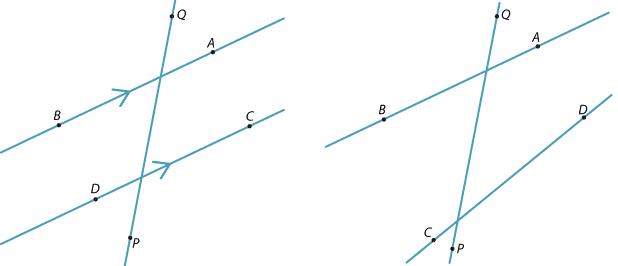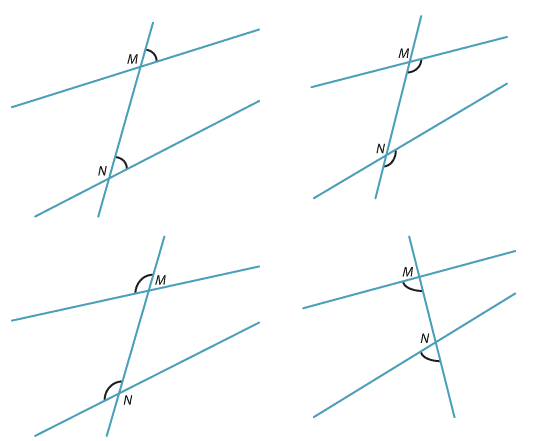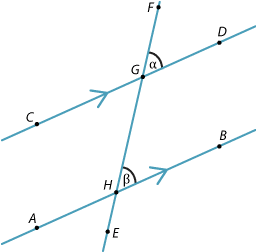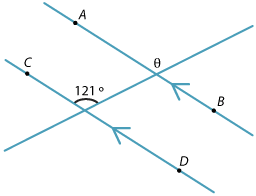Transversals

A transversal is a line that crosses two other lines. In both diagrams, the line PQ is a transversal to both the lines AB and CD.Corresponding angles

In each of the diagrams below, the two marked angles are called corresponding angles because they are in corresponding positions around the two vertices M and N.Detailed description

Corresponding angles and parallel lines

If the lines AB and CD are parallel, then the corresponding angles $$\angle$$DGF and $$\angle$$BHF, marked $$\alpha$$ and β, are equal.Detailed description

Example 1

Find θ in the diagram.Solution

θ = 121° (corresponding angles AB || CD)